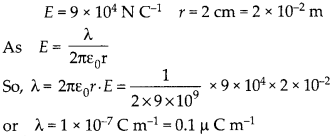Enlightened

# An infinite line charge produces a field of 9 × 104NC-1 at a distance of 2 cm. Calculate the linear charge density.

• 0

An infinite line charge produces a field of 9 × 104NC-1 at a distance of 2 cm. Calculate the linear charge density.

Share